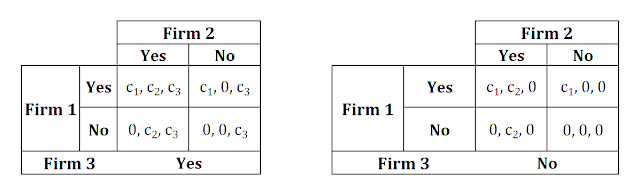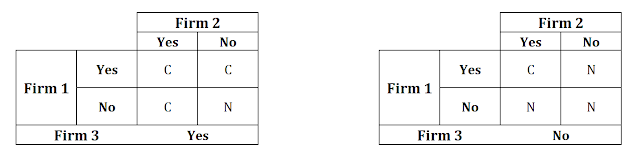# Real Income Trap: Is Economy Shrinking?

Real income remains how much money an individual or entity makes after accounting for inflation and is sometimes called real wage when referring to an individual's income. Overall, real income is an estimate of an individual’s purchasing power since the formula for calculating real income uses a broad collection of goods that may or may not closely match the categories an investor spends within. Purchasing power refers to what you are able to purchase, and it changes when your real income changes.

RI = W - W * Ir

=> RI = (1 - Ir)*W

where

• RI = Real Income
• W = Nominal Wage
• Ir = Inflation Rate

## Income Effect

The income effect is a change in the demand for a good or service due to a change in a consumer’s purchasing power, which is, in turn, due to a change in their real income. It’s part of the consumer choice economic theory that relates to how wealthy consumers feel. For example, when the price goes up the consumer is not able to buy as many products as he could purchase before. Can be easily understood that both firms and customers want real income to remain stable. Let RI_1, RI_2 the real income, W_1, W_2 the nominal wages and Ir_1,\ Ir_2 the inflation rate at the time period t_1 and t_2

RI_1 = RI_2

=> (1 - Ir_1)*W_1 = (1 - Ir_2)* W_2

=> W_2 / W_1 = (1 - Ir_1)/(1 - Ir_2)

=> W_2  = (1 - Ir_1)/(1 - Ir_2) * W_1

Also, let k = (1 - Ir_1)/(1 - Ir_2) != 0. Thus, the final equation is transformed to W_2 = k * W_1.

The real meaning of this equation has 3 dimensions:

Firstk = 1 <=> (1 - Ir_1) = (1 - Ir_2) <=> Ir_1 = Ir_2 <=> W_2 = W_1

Secondk < 1 <=> (1 - Ir_1) < (1 - Ir_2) <=> Ir_1 > Ir_2 <=> W_2 < W_1

Thirdk > 1<=> (1 - Ir_1) > (1 - Ir_2) <=> Ir_1 < Ir_2<=> W_2 > W_1

In other words, in a time period, in which the economy has high inflation the real income shrinks. The main solution to this bad situation is an increase in nominal wage. But is it possible? Every firm has two choices; increase the nominal wage, which can help the real income be stable, or not, which in the long run will shrink the demand and the economy. The cost of the increase for the firm i (i = 1, 2, 3,..., n) is c_i and the total cost is C = \sum_(i=1)^n c_i, which equals the sum of extra earnings for the employees - consumers.

## Game Theory Tools

Game theory deals with interactive situations where two or more individuals, called players, make decisions that jointly determine the final outcome. Let's consider the following game: During an inflation time period, there are only 3 firms in the economy. Each firm prefers to get maximum utility with the minimum cost. Maximum utility (U_(max)) is achieved, when in the long run demand remains at a high level. But for this achievement, employees' real income has to be stable over time.

ThusU_(max) <=> C = \sum_(i=1)^3 c_i

AlsoC \approx c_1 + c_2  and  C \approx c_2 + c_3  and  C \approx c_1 + c_3

AndC != c_1  or  C != c_2   or  C != c_3

In simple words, the economy will not be shrinking if on average real income is stable. That means, that is not necessary for all firms to increase the nominal wage, but enough of them have to.

1. Game-frame in strategic form:
• Players (I) =  {Firm 1, Firm 2. Firm 3}

• Strategies (S_i): {Yes (increase nominal wage, with cost c_i), No (stable nominal wage, with no extra cost)}; (S_1, S_2, S_3) = ({c_1, 0}, {c_2, 0}, {c_3, 0})

• Strategy Profiles (s) = {(c_1, c_2, c_3), (c_1, c_2, 0), (c_1,0 ,c_3), (0, c_2, c_3), (c_1, 0, 0), (0, 0, c_3), (0, c_2, 0), (0, 0, 0)}

• Outcomes (O): {C (high, stable demand in long run), N (shrinking demand in long run)}; outcomes are listed in Figure 2

• f : S \rightarrow O is a function that associates with every strategy profile s an outcome, f(s)\in O

• Utility: For every Player U_(max) = U(C) > U(N)

2. The graphic representation of the game is:Figure 1: Firm's Game [Own Processing]Figure 2: Firm's Game Outcomes [Own Processing]

In real life, every firm is selfish and greedy. It will make the decision, which will bring the desired utility at the lowest cost. In this firm's game, outcome C is strictly Pareto superior to N or, in terms of strategy profiles, ({c_1, c_2, c_3}, {c_1, c_2, 0}, {c_1,0 ,c_3}, {0, c_2, c_3}) are strictly Pareto superior to ({c_1, 0, 0}, {0, 0, c_3}, {0, c_2, 0}, {0, 0, 0}).

When a player has a strictly dominant strategy, it would be irrational to choose any other strategy, no matter what the other players do, because he would be guaranteed a lower payoff in every possible situation. If Firm 1 excepts that other firms will choose to increase nominal wages, it will choose to not, because in this strategy profile {0, c_2, c_3} the total Utility is U_(max) = U(C), without extra cost (c_1) for Firm 1. On the other hand, if Firm 1 excepts that other firms will not increase nominal wages will have not to increase it, because it is only a waste of money (U({c_1, 0, 0} = U(N) < U(C) = U_(max)). All firms follow the same logic.

As well, for every firm i, individual rationality leads to {0, 0, 0} despite the fact that both players would be better off if they both choose {c_1, c_2, c_3}It is obvious that if the firms could reach a binding agreement to increase nominal wage then they would do so; however, agreements are not possible, because of the huge number of firms in an economic sector. Also, any non-binding agreement will be a disaster, because if one firm expects the other firms to stick to the agreement, then it will cheat.

In conclusion, for every firm i, "No" strictly dominates "Yes" and s = {0,0,0} is a strictly dominant-strategy equilibrium, but in long run it will lead to an economic catastrophy.  In order to avoid this trap, an external body, like the state, has to force firms to increase nominal wages by at least Ir_t - Ir_(t-1) (k = 1 <=> W_2 = W_1).

## Selected References

Stefanos Stavrianos

Hello! I am a theoretical economist from Greece. Also, I have attended courses at Yale University, Stanford University, University of British Columbia and Higher School of Economics. If you want any help about the scientific area that I am working about, feel free to send me a message!

1.